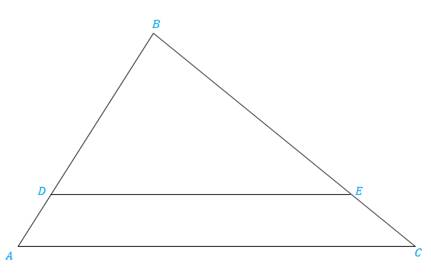Chapter 5.3, Problem 28EElementary Geometry For College St...

7th Edition
Alexander + 2 others
ISBN: 9781337614085

Solutions

Chapter
SectionElementary Geometry For College St...

7th Edition
Alexander + 2 others
ISBN: 9781337614085
Textbook Problem

In Exercises 25 to 28, Δ A B C ∼ Δ D B EExercises 25-28 Given: CB = 12, CE = 4, AD = 5. Find: DB

To determine

To find:

The side DB when the given sides are CB = 12, CE = 4, AD = 5 and ΔABCΔDBE.

Explanation

Definition:

CSSTP:

Corresponding sides of similar triangles are proportional.

Description:

The given figure is shown below.

Figure 1

From the given figure, it is observed that there are two triangles ABC and DBE and which is given that two triangles are similar.

Now draw the two triangles separately as shown below.

Figure 2

Figure 3

Since ΔABCΔDBE and from the definition of CSSTP, corresponding sides of similar triangles are proportional.

That is, BDBA=BEBC=DEAC.

It is given that CB = 12, CE = 4, AD = 5.

Find the side DB.

Consider the first two pairs of proportions BDBA=BEBC in order to find DB.

From Figure 1, it is observed that AB=AD+DB.

Find BE by using the given values

Still sussing out bartleby?

Check out a sample textbook solution.

See a sample solution

The Solution to Your Study Problems

Bartleby provides explanations to thousands of textbook problems written by our experts, many with advanced degrees!

Get Started

Find the 50th derivative of y = cos 2x.

Single Variable Calculus: Early Transcendentals, Volume I

In problems 9 and 10, which of and are subsets of? 10.

Mathematical Applications for the Management, Life, and Social Sciences

True or False: If f(x) 0 for all x in an interval I then f is increasing on I.

Study Guide for Stewart's Single Variable Calculus: Early Transcendentals, 8th